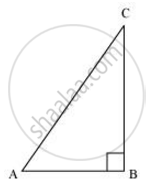# Given 15 cot A = 8. Find sin A and sec A - Mathematics

Given 15 cot A = 8. Find sin A and sec A

#### Solution

Consider a right-angled triangle, right-angled at B.cot A =(AB)/(BC)

= (AB)/(BC)

it is given that

cot A = 8/15

(AB)/(BC)=8/15

Let AB be 8k.Therefore, BC will be 15k, where k is a positive integer.

Applying Pythagoras theorem in ΔABC, we obtain

AC2 = AB2 + BC2

= (8k)2 + (15k)2

= 64k2 + 225k2

= 289k2

AC = 17k

sin A = (15k)/(17k) = 15/17

sec A  = (AC)/(AB) = 17/8

Is there an error in this question or solution?
Chapter 8: Introduction to Trigonometry - Exercise 8.1 [Page 181]

#### APPEARS IN

NCERT Class 10 Maths
Chapter 8 Introduction to Trigonometry
Exercise 8.1 | Q 4 | Page 181
RD Sharma Class 10 Maths
Chapter 10 Trigonometric Ratios
Exercise 10.1 | Q 5 | Page 24
Share